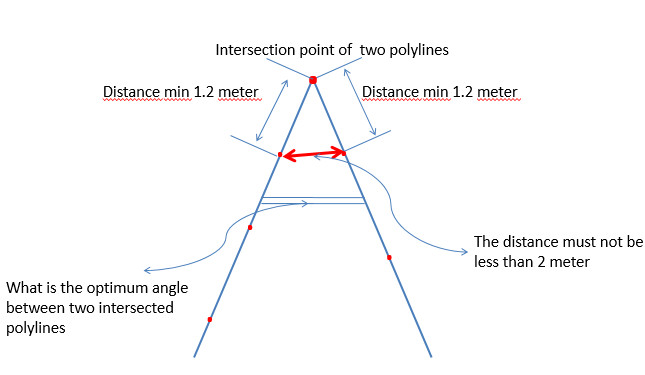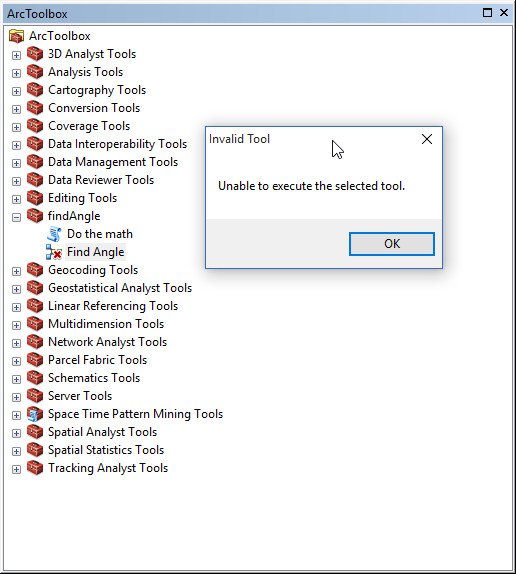# How to automatically calculate the angle between two intersecting polylines

14796
33
05-01-2016 02:43 PMOccasional Contributor II

Hello,

I have 52338 polygons which needs to be edited using the following rules;

The distance between the intersection point and the closest vertices on two  intersecting

polylines should be at least 1.2 meter as shown below. On the  other hand the distance

between two closest vertices should not be less than 2 meters. What I'm trying to do is

automatically calculate  the optimum angle angle between two polylines to meet the given

two rules as I explained above.ArcGIS Desktop doesn't have such built-in tool to calculate the angle as far as I know.

Is there any tool or script that you could suggest ?  If there is no such solution it would

also be great, to select vertices which are  2 meters apart from the intersection point.

Regards

Tags (2)
1 Solution

Accepted SolutionsbyMVP Legendary Contributor

Given the radius from the intersection point and the distances to the minimums on the intersecting lines, you have formed a chord Chord (geometry) - Wikipedia, the free encyclopedia

So you have the 'circle' radius, the chord distance (cd), hence the angle (theta in the link) can be solved as the minimum requirement given, r and cd.  As r varies so will theta, given a cd.

If you have already existing polylines, there is no guarantee that there will be vertices at r, so one will have to be created of course.

33 RepliesbyMVP Legendary ContributorbyMVP Legendary Contributor

Given the radius from the intersection point and the distances to the minimums on the intersecting lines, you have formed a chord Chord (geometry) - Wikipedia, the free encyclopedia

So you have the 'circle' radius, the chord distance (cd), hence the angle (theta in the link) can be solved as the minimum requirement given, r and cd.  As r varies so will theta, given a cd.

If you have already existing polylines, there is no guarantee that there will be vertices at r, so one will have to be created of course.Occasional Contributor II

Dan,

Thank you for the information about my question. I'm trying to figure out how I can apply the method

you summarized.byMVP Legendary Contributor

is it just the how to calculate angle part, or the whole process as perhaps shown in Wes's post, or have you not obtained the lines for calculating angles?Occasional Contributor II

Hello,

It is the whole process as shown on Wes's post.  I tried to run find angle tool but got error message

saying `unable to execute the selected tool`. I have ArcGIS  for Desktop 10.3.1 Advanced license.

Is there anything to fix this problem.byMVP Legendary Contributor

I have never used it, perhaps Wes Miller​ knows the ins and outs of itRegular Contributor IIIOccasional Contributor II

I checked the thread you're referring to. I'm using ArcGIS for Desktop Advanced (10.3.1) but I'm unable to run find angle tool  since ArcGIS 10.3.1 says `unable to execute the selected tool`. Is there a workaround for this issue ?Regular Contributor III

Right click the Find Angle tool and choose edit when the model opens change "Roads" to your line file. Then click "Model" -> "Run Entire Model" tell me where the process stops everything that has ran will have a shadow behind it.